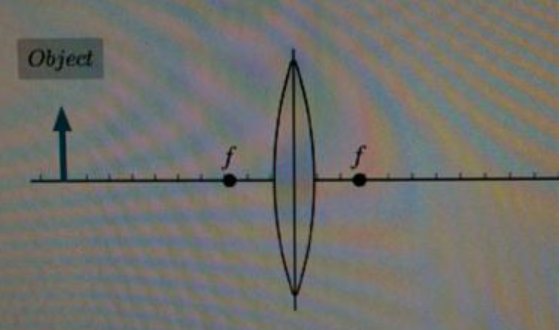# Problem: Learning Goal: To practice Tactics Box 23.2 Ray Tracing for a Converging Lens.The procedure known as ray tracing is a pictorial method for understanding image formation when lenses or mirrors are used. It consists of locating the image by the use of just three "special rays." The following tactics box explains this procedure for the case of a converging lens.TACTICS BOX 23.2 Ray tracing for a converging lensDraw an optical axis. Use graph paper or a ruler. Establish an appropriate scale.Center the lens on the axis. Mark and label the focal points at distance f on either side.Represent the object with an upright arrow at distance s'. It is usually best to place the base of the arrow on the axis and to draw the arrow about half the radius of the lens.Draw the three "special rays" from the tip of the arrow. Use a straightedge.A ray parallel to the axis (Ray 1) refracts through the far focal point.A ray that enters the lens along a line through the near focal point (Ray 2) emerges parallel to the axis.A ray through the center of the lens (Ray 3) does not bend.Extend the rays until they converge. This is the image point. Draw the rest of the image in the image plane. If the base of the object is on the axis, then the base of the image will also be on the axis.Measure the image distance s'. Also, if needed, measure the image height relative to the object height.Follow the steps above to solve the following problem: An object is 9.0 cm from a converging lens with a focal length of 2.8 cm. Use ray tracing to determine the location of the image.Part AThe diagram below shows the situation described in the problem. The focal length of the lens is labeled f; the scale on the optical axis is in centimeters.Draw the three special rays, Ray 1, Ray 2, and Ray 3, as described in the tactics box above, and label each ray accordingly. Do not draw the refracted rays.Draw the rays from the tip of the object to the lens.

###### FREE Expert Solution

The three special rays are:

1. A ray parallel to the axis (Ray 1)
2. A ray that enters the lens along a line through the near focal point (Ray 2)
3. A ray through the center of the lens (Ray 3)
88% (183 ratings)###### Problem Details

Learning Goal: To practice Tactics Box 23.2 Ray Tracing for a Converging Lens.

The procedure known as ray tracing is a pictorial method for understanding image formation when lenses or mirrors are used. It consists of locating the image by the use of just three "special rays." The following tactics box explains this procedure for the case of a converging lens.

TACTICS BOX 23.2 Ray tracing for a converging lens

1. Draw an optical axis. Use graph paper or a ruler. Establish an appropriate scale.
2. Center the lens on the axis. Mark and label the focal points at distance f on either side.
3. Represent the object with an upright arrow at distance s'. It is usually best to place the base of the arrow on the axis and to draw the arrow about half the radius of the lens.
4. Draw the three "special rays" from the tip of the arrow. Use a straightedge.
1. A ray parallel to the axis (Ray 1) refracts through the far focal point.
2. A ray that enters the lens along a line through the near focal point (Ray 2) emerges parallel to the axis.
3. A ray through the center of the lens (Ray 3) does not bend.
5. Extend the rays until they converge. This is the image point. Draw the rest of the image in the image plane. If the base of the object is on the axis, then the base of the image will also be on the axis.
6. Measure the image distance s'. Also, if needed, measure the image height relative to the object height.

Follow the steps above to solve the following problem: An object is 9.0 cm from a converging lens with a focal length of 2.8 cm. Use ray tracing to determine the location of the image.

Part A

The diagram below shows the situation described in the problem. The focal length of the lens is labeled f; the scale on the optical axis is in centimeters.

Draw the three special rays, Ray 1, Ray 2, and Ray 3, as described in the tactics box above, and label each ray accordingly. Do not draw the refracted rays.

Draw the rays from the tip of the object to the lens.